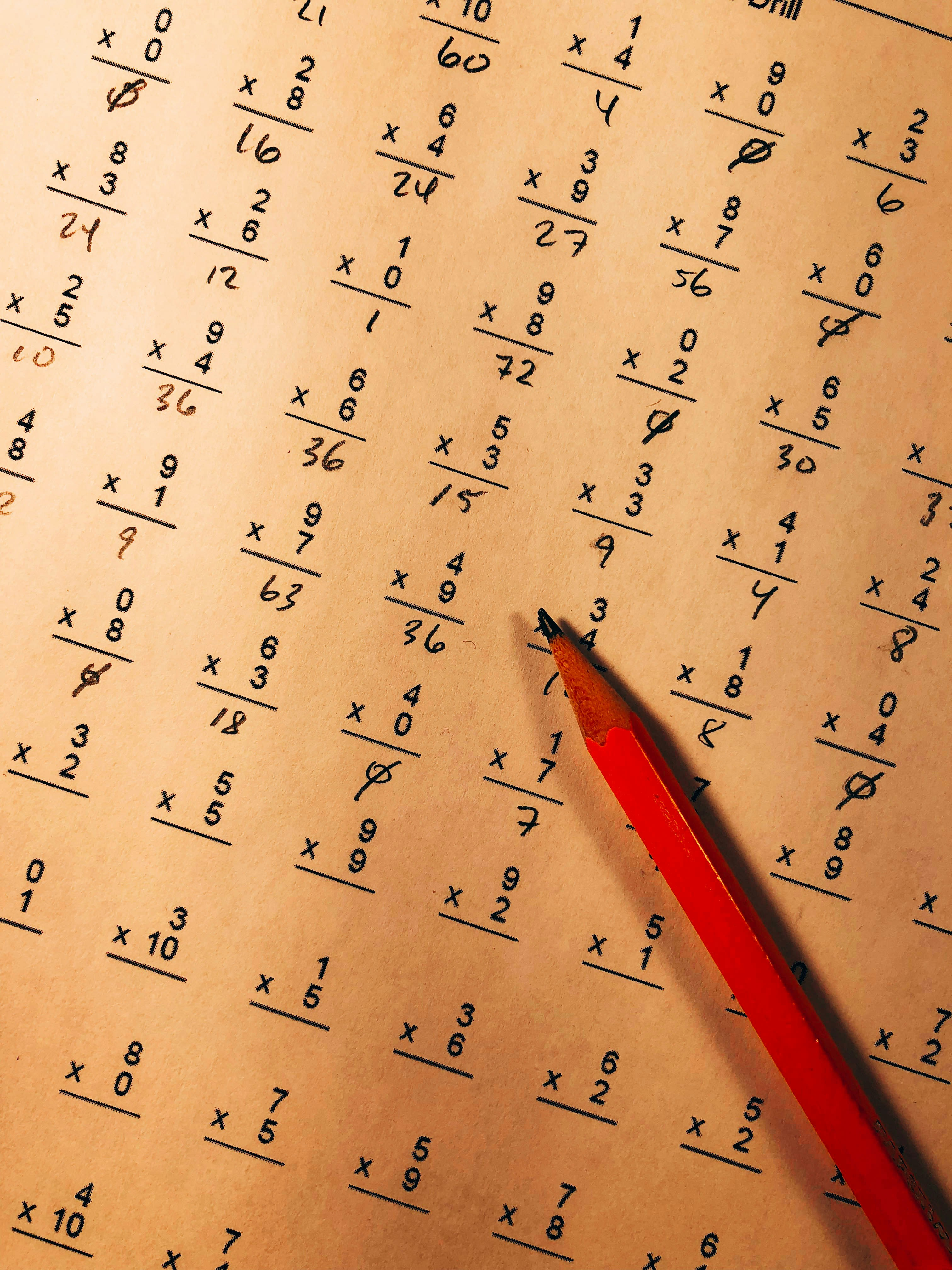# Arithmetic pictures

1 free arithmetic picturesred pencil on top of mathematical quiz paperred pencil on top of mathematical quiz paperred pencil on top of mathematical quiz paper

Make something awesome